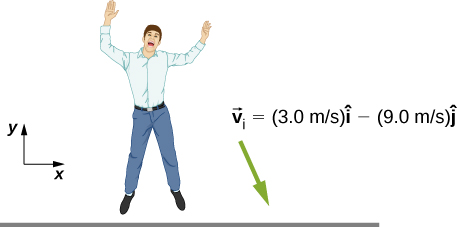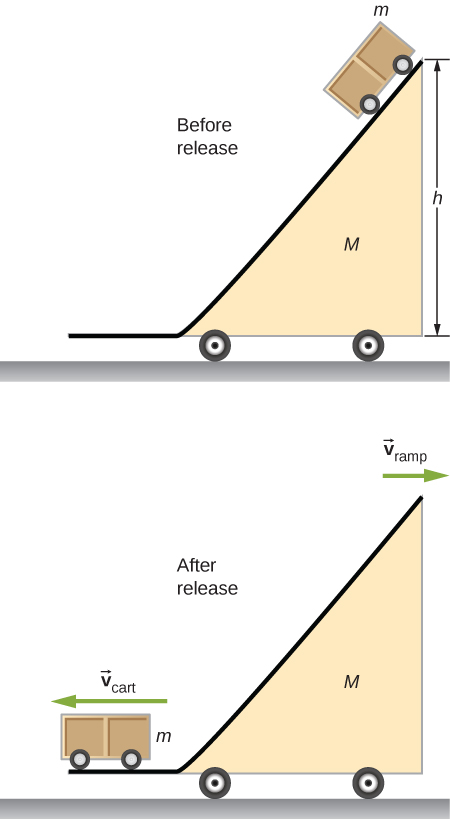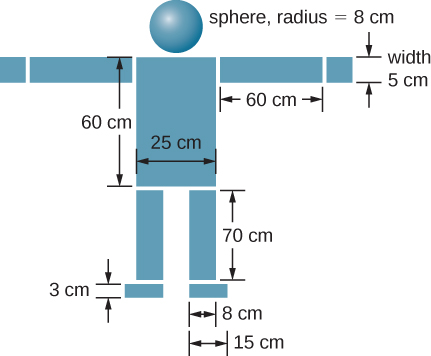9.7 Rocket propulsion  (Page 7/8)

 Page 7 / 8

Two billiard balls are at rest and touching each other on a pool table. The cue ball travels at 3.8 m/s along the line of symmetry between these balls and strikes them simultaneously. If the collision is elastic, what is the velocity of the three balls after the collision?

final velocity of cue ball is $\text{−}\left(0.76\phantom{\rule{0.2em}{0ex}}\text{m/s}\right)\stackrel{^}{i}$ , final velocities of the other two balls are 2.6 m/s at ±30° with respect to the initial velocity of the cue ball

A billiard ball traveling at $\left(2.2\phantom{\rule{0.2em}{0ex}}\text{m/s}\right)\stackrel{^}{i}-\left(0.4\phantom{\rule{0.2em}{0ex}}\text{m/s}\right)\stackrel{^}{j}$ collides with a wall that is aligned in the $\stackrel{^}{j}$ direction. Assuming the collision is elastic, what is the final velocity of the ball?

Two identical billiard balls collide. The first one is initially traveling at $\left(2.2\phantom{\rule{0.2em}{0ex}}\text{m/s}\right)\stackrel{^}{i}-\left(0.4\phantom{\rule{0.2em}{0ex}}\text{m/s}\right)\stackrel{^}{j}$ and the second one at $\text{−}\left(1.4\phantom{\rule{0.2em}{0ex}}\text{m/s}\right)\stackrel{^}{i}+\left(2.4\phantom{\rule{0.2em}{0ex}}\text{m/s}\right)\stackrel{^}{j}$ . Suppose they collide when the center of ball 1 is at the origin and the center of ball 2 is at the point $\left(2R,0\right)$ where R is the radius of the balls. What is the final velocity of each ball?

ball 1: $\text{−}\left(1.4\phantom{\rule{0.2em}{0ex}}\text{m/s}\right)\stackrel{^}{i}-\left(0.4\phantom{\rule{0.2em}{0ex}}\text{m/s}\right)\stackrel{^}{j}$ , ball 2: $\left(2.2\phantom{\rule{0.2em}{0ex}}\text{m/s}\right)\stackrel{^}{i}+\left(2.4\phantom{\rule{0.2em}{0ex}}\text{m/s}\right)\stackrel{^}{j}$

Repeat the preceding problem if the balls collide when the center of ball 1 is at the origin and the center of ball 2 is at the point $\left(0,2R\right)$ .

Repeat the preceding problem if the balls collide when the center of ball 1 is at the origin and the center of ball 2 is at the point $\left(\sqrt{3}R\text{/}2,R\text{/}2\right)$

ball 1: $\left(1.4\phantom{\rule{0.2em}{0ex}}\text{m/s}\right)\stackrel{^}{i}-\left(1.7\phantom{\rule{0.2em}{0ex}}\text{m/s}\right)\stackrel{^}{j}$ , ball 2: $\text{−}\left(2.8\phantom{\rule{0.2em}{0ex}}\text{m/s}\right)\stackrel{^}{i}+\left(0.012\phantom{\rule{0.2em}{0ex}}\text{m/s}\right)\stackrel{^}{j}$

Where is the center of mass of a semicircular wire of radius R that is centered on the origin, begins and ends on the x axis, and lies in the x , y plane?

Where is the center of mass of a slice of pizza that was cut into eight equal slices? Assume the origin is at the apex of the slice and measure angles with respect to an edge of the slice. The radius of the pizza is R .

$\left(r,\theta \right)=\left(2R\text{/}3,\pi \text{/}8\right)$

If the entire population of Earth were transferred to the Moon, how far would the center of mass of the Earth-Moon-population system move? Assume the population is 7 billion, the average human has a mass of 65 kg, and that the population is evenly distributed over both the Earth and the Moon. The mass of the Earth is $5.97\phantom{\rule{0.2em}{0ex}}×\phantom{\rule{0.2em}{0ex}}{10}^{24}\text{kg}$ and that of the Moon is $7.34\phantom{\rule{0.2em}{0ex}}×\phantom{\rule{0.2em}{0ex}}{10}^{22}\text{kg}$ . The radius of the Moon’s orbit is about $3.84\phantom{\rule{0.2em}{0ex}}×\phantom{\rule{0.2em}{0ex}}{10}^{5}\text{m}$ .

You friend wonders how a rocket continues to climb into the sky once it is sufficiently high above the surface of Earth so that its expelled gasses no longer push on the surface. How do you respond?

Answers may vary. The rocket is propelled forward not by the gasses pushing against the surface of Earth, but by conservation of momentum. The momentum of the gas being expelled out the back of the rocket must be compensated by an increase in the forward momentum of the rocket.

To increase the acceleration of a rocket, should you throw rocks out of the front window of the rocket or out of the back window?

Challenge

A 65-kg person jumps from the first floor window of a burning building and lands almost vertically on the ground with a horizontal velocity of 3 m/s and vertical velocity of $-9\phantom{\rule{0.2em}{0ex}}\text{m/s}$ . Upon impact with the ground he is brought to rest in a short time. The force experienced by his feet depends on whether he keeps his knees stiff or bends them. Find the force on his feet in each case.1. First find the impulse on the person from the impact on the ground. Calculate both its magnitude and direction.
2. Find the average force on the feet if the person keeps his leg stiff and straight and his center of mass drops by only 1 cm vertically and 1 cm horizontally during the impact.
3. Find the average force on the feet if the person bends his legs throughout the impact so that his center of mass drops by 50 cm vertically and 5 cm horizontally during the impact.
4. Compare the results of part (b) and (c), and draw conclusions about which way is better.

You will need to find the time the impact lasts by making reasonable assumptions about the deceleration. Although the force is not constant during the impact, working with constant average force for this problem is acceptable.

a. $617\phantom{\rule{0.2em}{0ex}}\text{N}·\text{s}$ , 108°; b. ${F}_{x}=2.91\phantom{\rule{0.2em}{0ex}}×\phantom{\rule{0.2em}{0ex}}{10}^{4}\phantom{\rule{0.2em}{0ex}}\text{N}$ , ${F}_{y}=2.6\phantom{\rule{0.2em}{0ex}}×\phantom{\rule{0.2em}{0ex}}{10}^{5}\phantom{\rule{0.2em}{0ex}}\text{N}$ ; c. ${F}_{x}=5265\phantom{\rule{0.2em}{0ex}}\text{N}$ , ${F}_{y}=5850\phantom{\rule{0.2em}{0ex}}\text{N}$

Two projectiles of mass ${m}_{1}$ and ${m}_{2}$ are ﬁred at the same speed but in opposite directions from two launch sites separated by a distance D . They both reach the same spot in their highest point and strike there. As a result of the impact they stick together and move as a single body afterwards. Find the place they will land.

Two identical objects (such as billiard balls) have a one-dimensional collision in which one is initially motionless. After the collision, the moving object is stationary and the other moves with the same speed as the other originally had. Show that both momentum and kinetic energy are conserved.

Conservation of momentum demands ${m}_{1}{v}_{\text{1,i}}+{m}_{2}{v}_{\text{2,i}}={m}_{1}{v}_{\text{1,f}}+{m}_{2}{v}_{\text{2,f}}$ . We are given that ${m}_{1}={m}_{2}$ , ${v}_{\text{1,i}}={v}_{\text{2,f}}$ , and ${v}_{\text{2,i}}={v}_{\text{1,f}}=0$ . Combining these equations with the equation given by conservation of momentum gives ${v}_{\text{1,i}}={v}_{\text{1,i}}$ , which is true, so conservation of momentum is satisfied. Conservation of energy demands $\frac{1}{2}{m}_{1}{v}_{\text{1,i}}^{2}+\frac{1}{2}{m}_{2}{v}_{\text{2,i}}^{2}=\frac{1}{2}{m}_{1}{v}_{\text{1,f}}^{2}+\frac{1}{2}{m}_{2}{v}_{\text{2,f}}^{2}$ . Again combining this equation with the conditions given above give ${v}_{\text{1,i}}={v}_{\text{1,i}}$ , so conservation of energy is satisfied.

A ramp of mass M is at rest on a horizontal surface. A small cart of mass m is placed at the top of the ramp and released.What are the velocities of the ramp and the cart relative to the ground at the instant the cart leaves the ramp?

Find the center of mass of the structure given in the figure below. Assume a uniform thickness of 20 cm, and a uniform density of $1{\phantom{\rule{0.2em}{0ex}}\text{g/cm}}^{3}.$Assume origin on centerline and at floor, then $\left({x}_{\text{CM}},{y}_{\text{CM}}\right)=\left(0,86\phantom{\rule{0.2em}{0ex}}\text{cm}\right)$

a particle projected from origin moving on x-y plane passes through P & Q having consituents (9,7) , (18,4) respectively.find eq. of trajectry.
definition of inertia
the reluctance of a body to start moving when it is at rest and to stop moving when it is in motion
charles
An inherent property by virtue of which the body remains in its pure state or initial state
Kushal
why current is not a vector quantity , whereas it have magnitude as well as direction.
why
daniel
the flow of current is not current
fitzgerald
bcoz it doesn't satisfy the algabric laws of vectors
Shiekh
The Electric current can be defined as the dot product of the current density and the differential cross-sectional area vector : ... So the electric current is a scalar quantity . Scalars are related to tensors by the fact that a scalar is a tensor of order or rank zero .
Kushal
what is binomial theorem
what is binary operations
Tollum
What is the formula to calculat parallel forces that acts in opposite direction?
position, velocity and acceleration of vector
hi
peter
hi
daniel
hi
Vedisha
*a plane flies with a velocity of 1000km/hr in a direction North60degree east.find it effective velocity in the easterly and northerly direction.*
imam
hello
Lydia
hello Lydia.
Sackson
What is momentum
isijola
hello
A rail way truck of mass 2400kg is hung onto a stationary trunk on a level track and collides with it at 4.7m|s. After collision the two trunk move together with a common speed of 1.2m|s. Calculate the mass of the stationary trunk
I need the solving for this question
philip
is the eye the same like the camera
I can't understand
Suraia
Josh
I think the question is that ,,, the working principal of eye and camera same or not?
Sardar
yes i think is same as the camera
what are the dimensions of surface tension
samsfavor
why is the "_" sign used for a wave to the right instead of to the left?
why classical mechanics is necessary for graduate students?
classical mechanics?
Victor
principle of superposition?
principle of superposition allows us to find the electric field on a charge by finding the x and y components
Kidus
Two Masses,m and 2m,approach each along a path at right angles to each other .After collision,they stick together and move off at 2m/s at angle 37° to the original direction of the mass m. What where the initial speeds of the two particles
MB
2m & m initial velocity 1.8m/s & 4.8m/s respectively,apply conservation of linear momentum in two perpendicular directions.
Shubhrant
A body on circular orbit makes an angular displacement given by teta(t)=2(t)+5(t)+5.if time t is in seconds calculate the angular velocity at t=2s
MB
2+5+0=7sec differentiate above equation w.r.t time, as angular velocity is rate of change of angular displacement.
Shubhrant
Ok i got a question I'm not asking how gravity works. I would like to know why gravity works. like why is gravity the way it is. What is the true nature of gravity?
gravity pulls towards a mass...like every object is pulled towards earth
Ashok
An automobile traveling with an initial velocity of 25m/s is accelerated to 35m/s in 6s,the wheel of the automobile is 80cm in diameter. find * The angular acceleration
(10/6) ÷0.4=4.167 per sec
Shubhrant
what is the formula for pressure?
force/area
Kidus
force is newtom
Kidus
and area is meter squared
Kidus
so in SI units pressure is N/m^2
Kidus
In customary United States units pressure is lb/in^2. pound per square inch
KidusBy Lakeima RobertsBy Jordon Humphreys